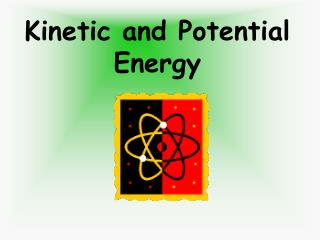Download PresentationKinetic and Potential Energy

# Kinetic and Potential Energy

Download Presentation## Kinetic and Potential Energy

- - - - - - - - - - - - - - - - - - - - - - - - - - - E N D - - - - - - - - - - - - - - - - - - - - - - - - - - -
##### Presentation Transcript

1. Kinetic and Potential Energy

2. After the Lesson: • You will be able to define and identify Kinetic and Potential energy. • You will be able to give examples of the two forms of energy. • You will be able to explain how one can transfer to the other.

3. Definition of Energy… The ability or capacity to do work. Measured by the capability of doing work: potential energy or the conversion of this capability to motion: kinetic energy.

4. Types of Energy Kinetic Energy Potential Energy

5. Forms of Energy Chemical Sound Radiant Electrical Mechanical Magnetic Thermal Nuclear

6. Potential Energy… • Energy due to position or stored energy. Potential energy is calculated by: The object’s weight, multiplied by the earth's gravitational pull (9.8 m/sec sq), multiplied by the distance the object can fall.

7. POTENTIAL ENERGY Potential energy exists whenever an object which has mass has a position within a force field. The most everyday example of this is the position of objects in the earth's gravitational field. The potential energy of an object in this case is given by the relation: PE = mgh  PE = Energy (in Joules) m = mass (in kilograms) g = gravitational acceleration of the earth (9.8 m/sec2) h = height above earth's surface (in meters)

8. Examples of Potential Energy: Stretching a rubber band.. -Stores energy Water at the top of a waterfall.. -Stores energy Yo–Yo in held in your hand.. -Stores energy because of position Drawing a Bow… -Stores energy because of position

9. When the position of an object is altered it, creates Potential Energy. • A yo-yo on the table, doesn’t have energy, but when picked up, it alters its position and now it has the ability (or potential) to do work. • A bow doesn’t have the capacity to do work, unless it’s held at an elevated position.

10. Definition of Kinetic Energy… The energy of motion. Kinetic energy is calculated by one half of the object’s mass, multiplied by the object’s speed- squared.

11. KINETIC ENERGY The greater the mass or velocity of a moving object, the more kinetic energy it has. Kinetic Energy Lab

12. Examples of Kinetic Energy… • Shooting a rubber band. • Water falling over the fall. • A Yo-Yo in motion. • Releasing the arrow from the bow.

13. Potential Energy Converted to Kinetic Energy… When stored energy begins to move, the object now transfers from potential energy into kinetic energy. Standing still Running

14. What happens when the cord is cut? Potential energy is converted to kinetic energy!

15. Potential Energy- • “stored” energy • related to an object’s height above the ground • the higher something is, the more potential energy it has • Kinetic Energy- • “energy of motion” • related to an object’s velocity • the faster something is traveling, the more kinetic energy it has

16. Directions • Decide whether each slide is an example of potential or kinetic energy. • Click on your answer to see if you are correct. Whenever you see this picture, you will need to write the answer in your journal.

17. The Ball Kinetic Energy Potential Energy

18. The Ball Kinetic Energy Potential Energy

19. Potential Energy Kinetic Energy

20. Potential Energy Kinetic Energy

21. Kinetic Energy Potential Energy

22. Kinetic Energy Potential Energy

23. Potential Energy Kinetic Energy

24. Potential Energy Kinetic Energy

25. Kinetic Energy Potential Energy

26. Kinetic Energy Potential Energy

27. Conservation of energy says that the amount of energy the coaster has will always be constant. This means the potential energy of the car plus the kinetic energy of the car must always be the same. If the potential goes up, the kinetic must come down; if the kinetic goes up, the potential must come down.

28. The Hill: Conservation of Energy Why is the first hill of the roller coaster always the highest?

29. At the top of the first hill: • Kinetic Energy? • The coaster’s velocity is zero . . . • Kinetic energy = 0 • Potential Energy? • The coaster is very high . . . • Potential energy = high • All of the coaster’s energy is in the form of potential energy.

30. At the bottom of the hill: • Kinetic Energy? • The coaster is moving at a high velocity. • Kinetic energy = high • Potential Energy? • The height of the coaster is zero . . . • Potential energy = 0 • By the time the coaster reaches the bottom of the hill, all potential energy has been transformed to kinetic energy.

31. But why is the first hill highest? • When the coaster reaches the bottom of the first hill, all its energy has been transformed from potential to kinetic energy. • As it goes up the next hill, that kinetic energy must be transformed back into potential energy so the process can repeat. • But don’t forget friction – the coaster is always losing energy to friction between the car and the tracks, so each time it goes up a hill it will have less kinetic energy to transform back into potential.

32. The first hill of a roller coaster always must be the highest, otherwise the coaster won’t have enough energy to get up the other hills.